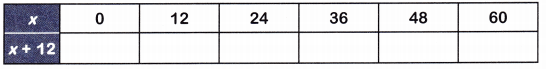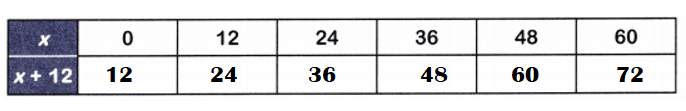Practice the questions of McGraw Hill Math Grade 4 Answer Key PDF Chapter 2 Lesson 6 Addition and Subtraction Patterns to secure good marks & knowledge in the exams.

Add or subtract to complete each table
Question 1.Explanation:
y = 0
=> y + 5 = 0 + 5 = 5.
y = 1
=> y + 5 = 1 + 5 = 6.
y = 2
=> y + 5 = 2 + 5 = 7.
y = 3
=> y + 5 = 3 + 5 = 8.

Question 2.Explanation:
x = 0
=> x + 12 = 0 + 12 = 12.
x = 12
=> x + 12 = 12 + 12 = 24.
x = 24
=> x + 12 = 24 + 12 = 36.
x = 36
=> x + 12 = 36 + 12 = 48.
x = 48
=> x + 12 = 48 + 12 = 60.
x = 60
=> x + 12 = 60 + 12 = 72.

Question 3.Explanation:
a = 30
=> a – 3 = 30 – 3 = 27.
a = 27
=> a – 3 = 27 – 3 = 24.
a = 24
=> a – 3 = 24 – 3 = 21.
a = 21
=> a – 3 = 21 – 3 = 18.
a = 18
=> a – 3 = 18 – 3 = 15.
a = 15
=> a – 3 = 15 – 3 = 12.

Question 4.
Look at problem 2. What does x + 12 mean?